Скачать презентацию Managing Interest Rate Risk II Duration GAP and Economic

64f5d1474e2745f8a466f0189ef86b2d.ppt

• Количество слайдов: 38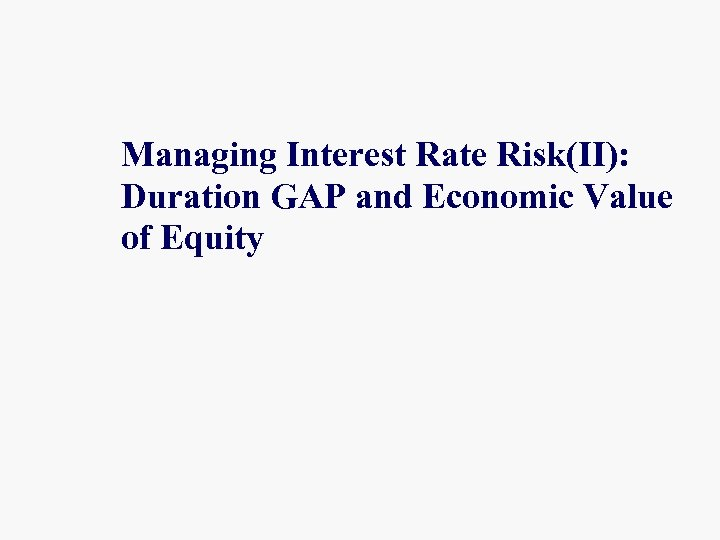Managing Interest Rate Risk(II): Duration GAP and Economic Value of Equity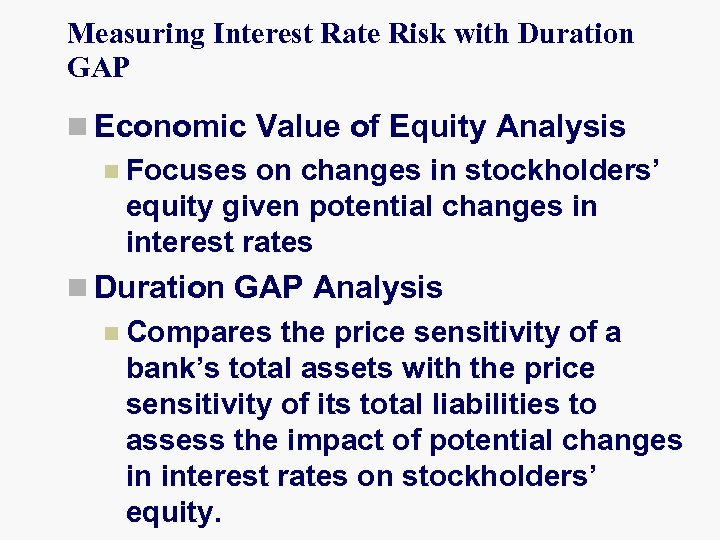Measuring Interest Rate Risk with Duration GAP n Economic Value of Equity Analysis n Focuses on changes in stockholders’ equity given potential changes in interest rates n Duration GAP Analysis n Compares the price sensitivity of a bank’s total assets with the price sensitivity of its total liabilities to assess the impact of potential changes in interest rates on stockholders’ equity.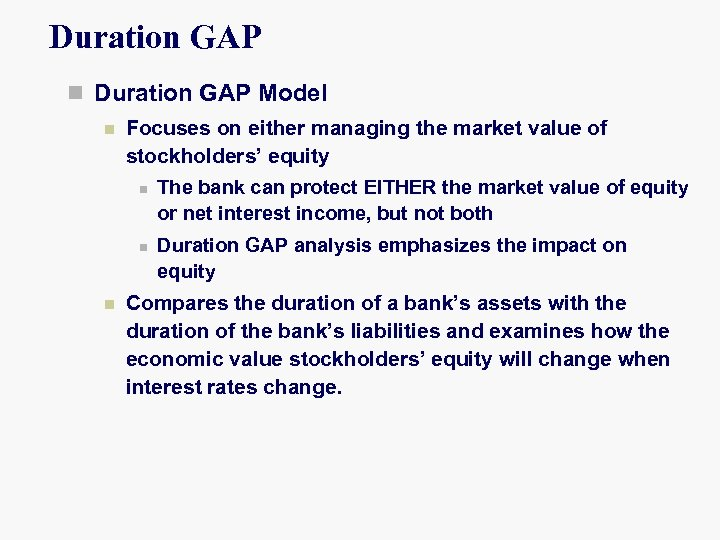Duration GAP n Duration GAP Model n Focuses on either managing the market value of stockholders’ equity n The bank can protect EITHER the market value of equity or net interest income, but not both n Duration GAP analysis emphasizes the impact on equity n Compares the duration of a bank’s assets with the duration of the bank’s liabilities and examines how the economic value stockholders’ equity will change when interest rates change.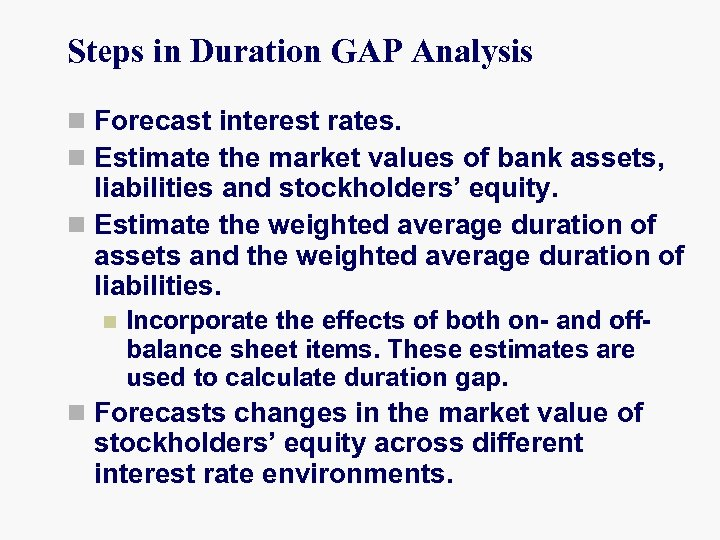Steps in Duration GAP Analysis n Forecast interest rates. n Estimate the market values of bank assets, liabilities and stockholders’ equity. n Estimate the weighted average duration of assets and the weighted average duration of liabilities. n Incorporate the effects of both on- and offbalance sheet items. These estimates are used to calculate duration gap. n Forecasts changes in the market value of stockholders’ equity across different interest rate environments.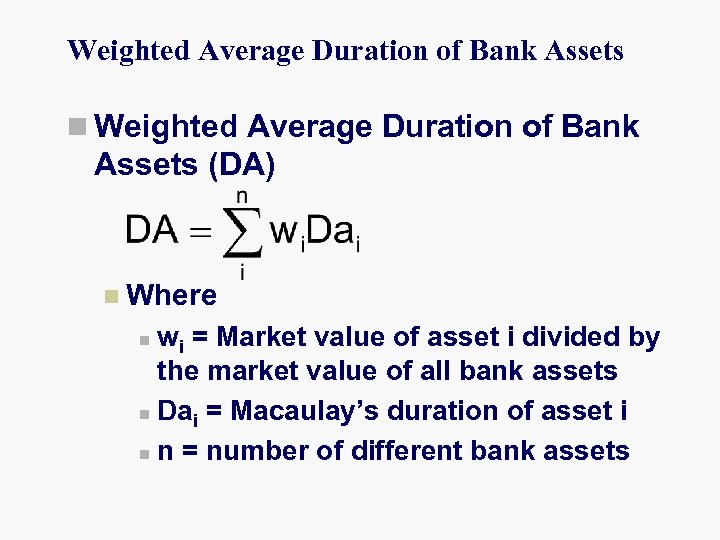Weighted Average Duration of Bank Assets n Weighted Average Duration of Bank Assets (DA) n Where wi = Market value of asset i divided by the market value of all bank assets n Dai = Macaulay’s duration of asset i n n = number of different bank assets n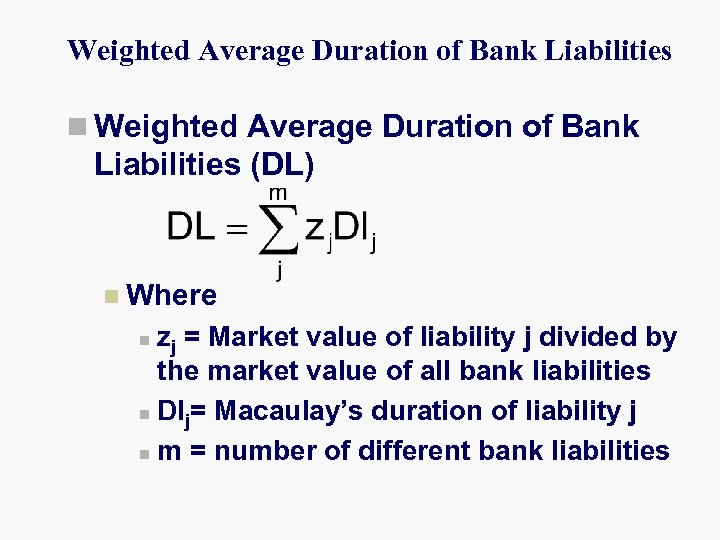Weighted Average Duration of Bank Liabilities n Weighted Average Duration of Bank Liabilities (DL) n Where zj = Market value of liability j divided by the market value of all bank liabilities n Dlj= Macaulay’s duration of liability j n m = number of different bank liabilities n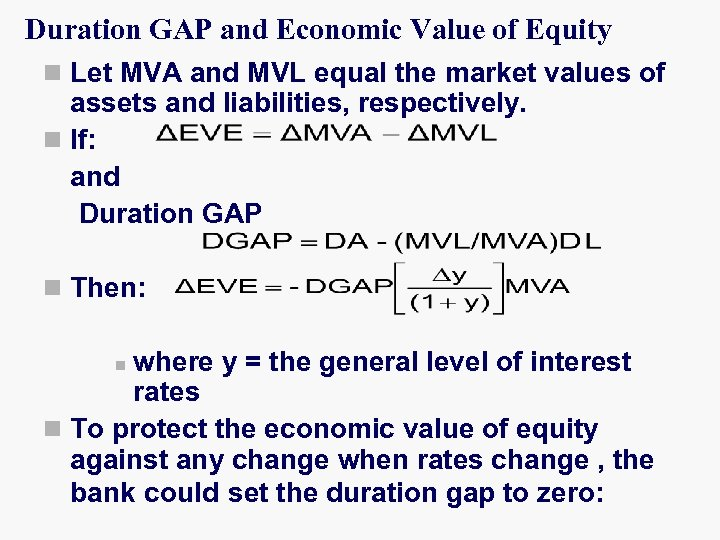Duration GAP and Economic Value of Equity n Let MVA and MVL equal the market values of assets and liabilities, respectively. n If: and Duration GAP n Then: where y = the general level of interest rates n To protect the economic value of equity against any change when rates change , the bank could set the duration gap to zero: n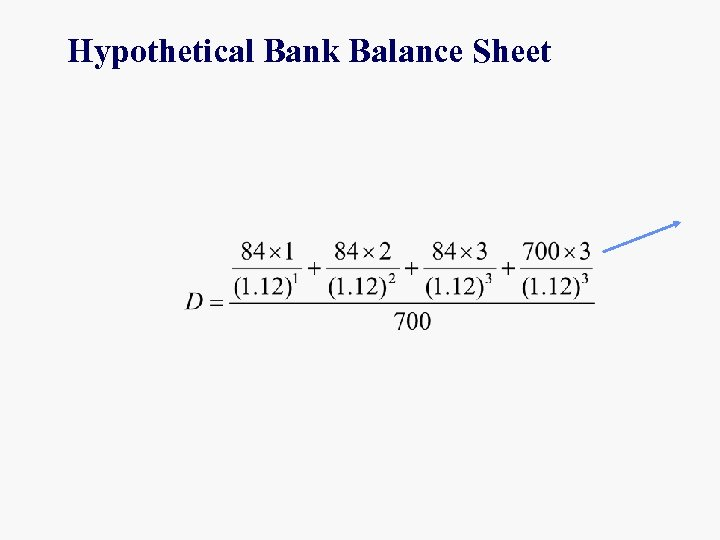Hypothetical Bank Balance Sheet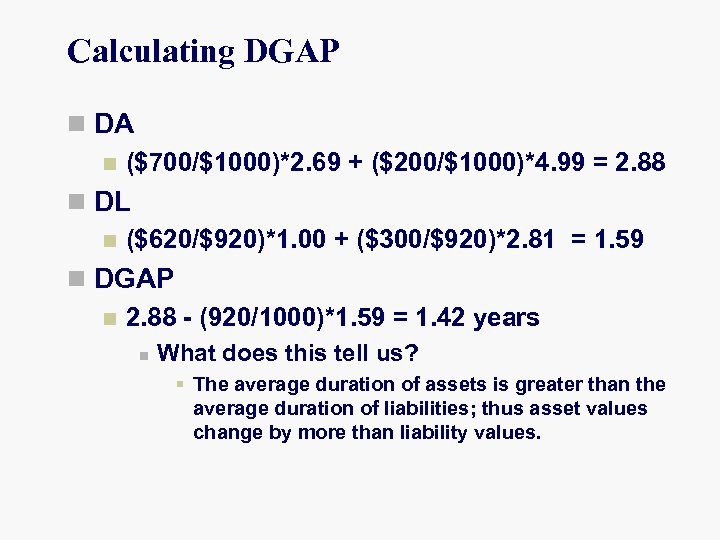Calculating DGAP n DA n (\$700/\$1000)*2. 69 + (\$200/\$1000)*4. 99 = 2. 88 n DL n (\$620/\$920)*1. 00 + (\$300/\$920)*2. 81 = 1. 59 n DGAP n 2. 88 - (920/1000)*1. 59 = 1. 42 years n What does this tell us? § The average duration of assets is greater than the average duration of liabilities; thus asset values change by more than liability values.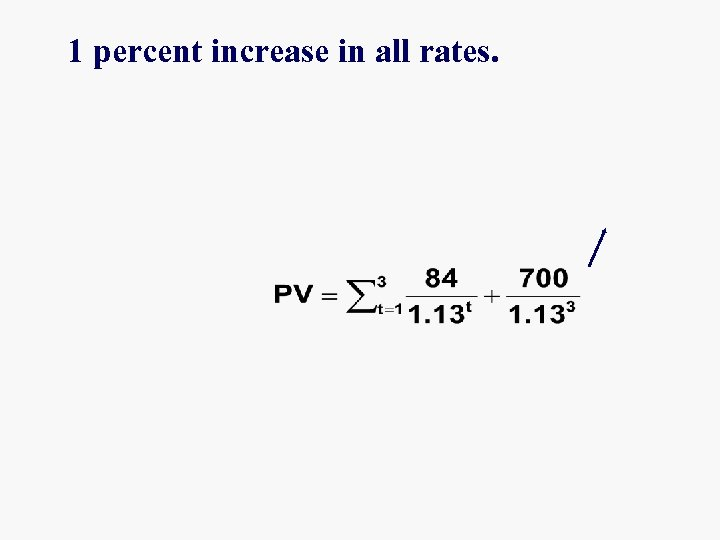1 percent increase in all rates.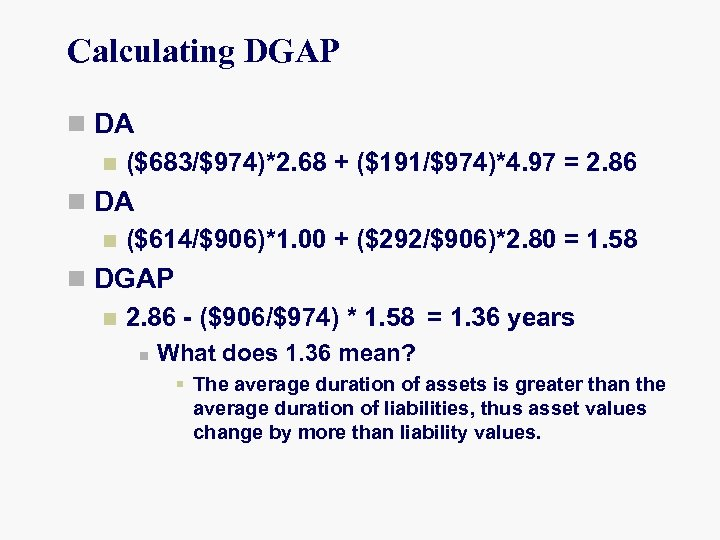Calculating DGAP n DA n (\$683/\$974)*2. 68 + (\$191/\$974)*4. 97 = 2. 86 n DA n (\$614/\$906)*1. 00 + (\$292/\$906)*2. 80 = 1. 58 n DGAP n 2. 86 - (\$906/\$974) * 1. 58 = 1. 36 years n What does 1. 36 mean? § The average duration of assets is greater than the average duration of liabilities, thus asset values change by more than liability values.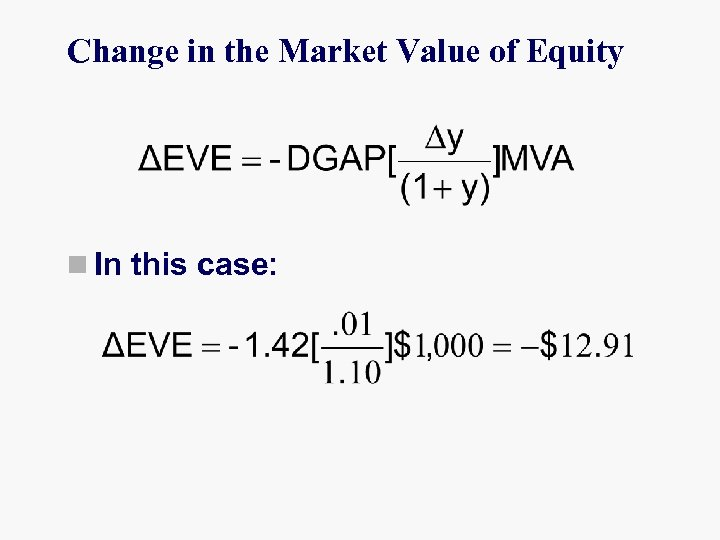Change in the Market Value of Equity n In this case: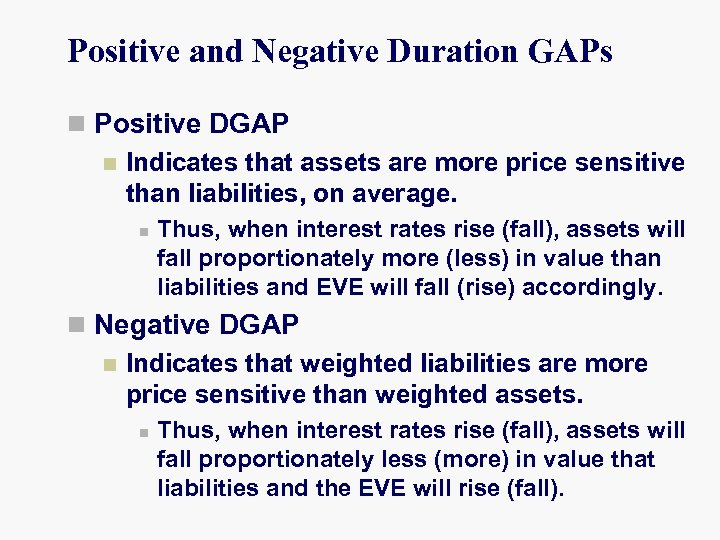Positive and Negative Duration GAPs n Positive DGAP n Indicates that assets are more price sensitive than liabilities, on average. n Thus, when interest rates rise (fall), assets will fall proportionately more (less) in value than liabilities and EVE will fall (rise) accordingly. n Negative DGAP n Indicates that weighted liabilities are more price sensitive than weighted assets. n Thus, when interest rates rise (fall), assets will fall proportionately less (more) in value that liabilities and the EVE will rise (fall).DGAP Summary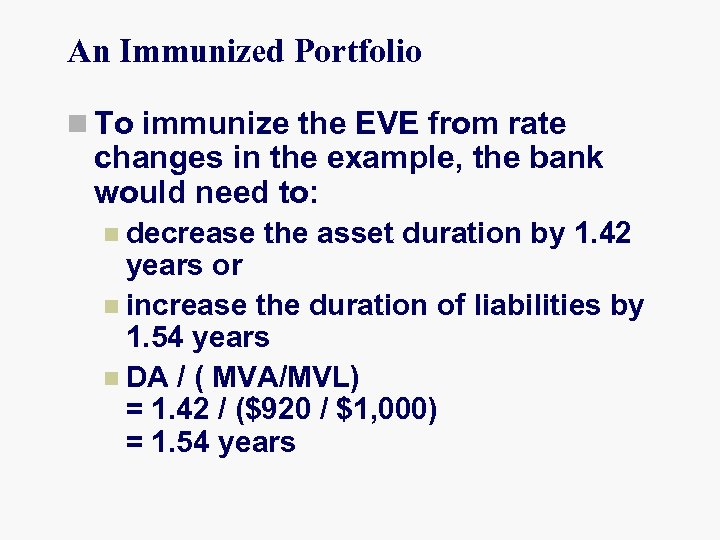An Immunized Portfolio n To immunize the EVE from rate changes in the example, the bank would need to: n decrease the asset duration by 1. 42 years or n increase the duration of liabilities by 1. 54 years n DA / ( MVA/MVL) = 1. 42 / (\$920 / \$1, 000) = 1. 54 years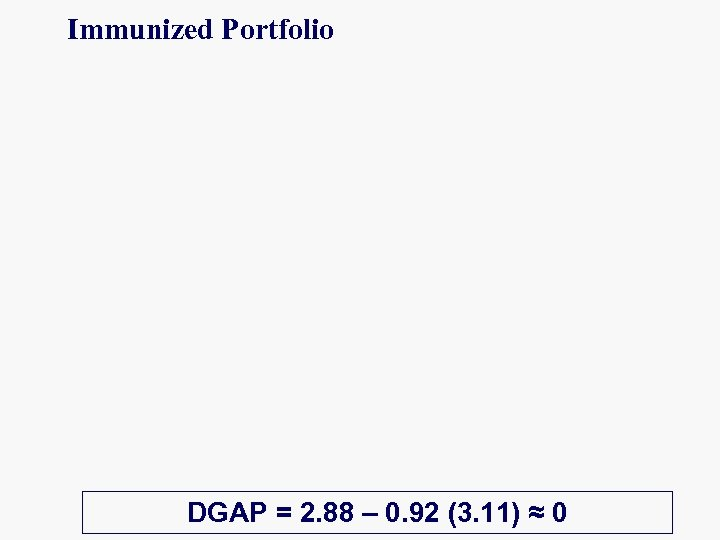Immunized Portfolio DGAP = 2. 88 – 0. 92 (3. 11) ≈ 0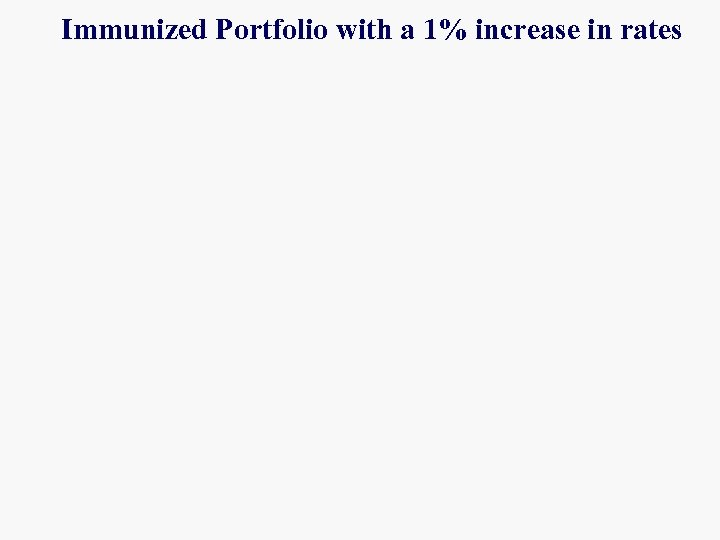Immunized Portfolio with a 1% increase in rates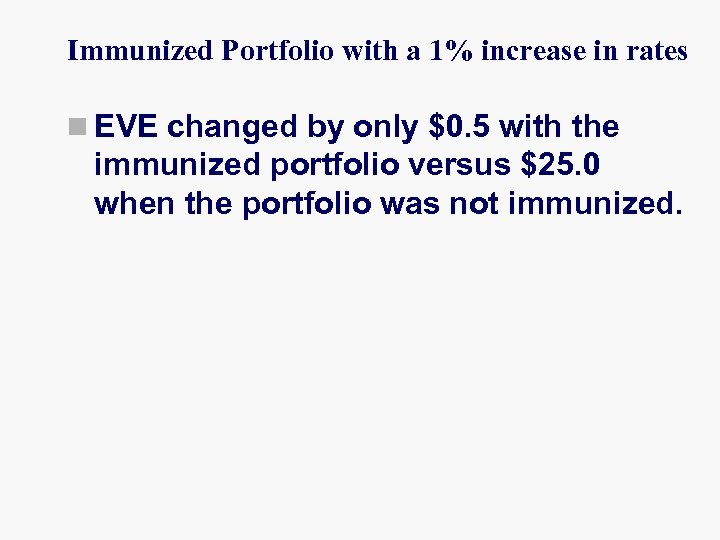Immunized Portfolio with a 1% increase in rates n EVE changed by only \$0. 5 with the immunized portfolio versus \$25. 0 when the portfolio was not immunized.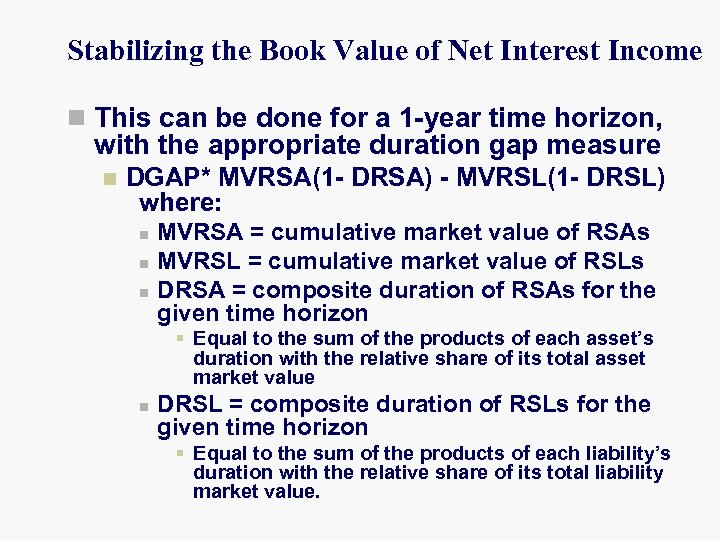Stabilizing the Book Value of Net Interest Income n This can be done for a 1 -year time horizon, with the appropriate duration gap measure n DGAP* MVRSA(1 - DRSA) - MVRSL(1 - DRSL) where: n n n MVRSA = cumulative market value of RSAs MVRSL = cumulative market value of RSLs DRSA = composite duration of RSAs for the given time horizon § Equal to the sum of the products of each asset’s duration with the relative share of its total asset market value n DRSL = composite duration of RSLs for the given time horizon § Equal to the sum of the products of each liability’s duration with the relative share of its total liability market value.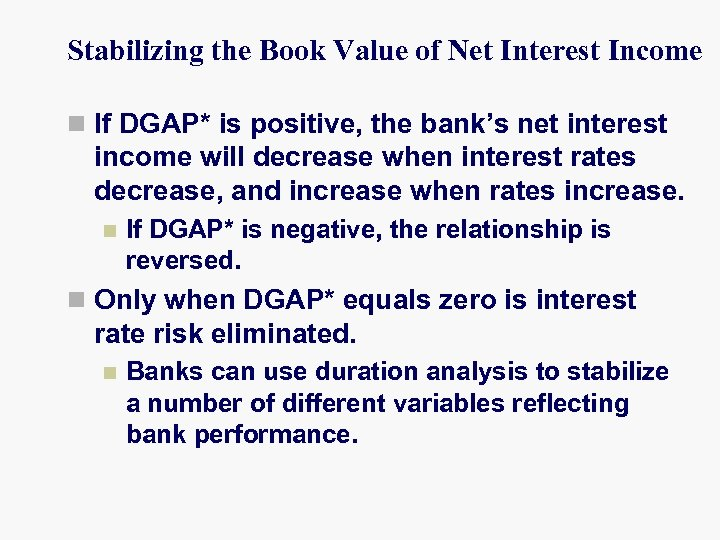Stabilizing the Book Value of Net Interest Income n If DGAP* is positive, the bank’s net interest income will decrease when interest rates decrease, and increase when rates increase. n If DGAP* is negative, the relationship is reversed. n Only when DGAP* equals zero is interest rate risk eliminated. n Banks can use duration analysis to stabilize a number of different variables reflecting bank performance.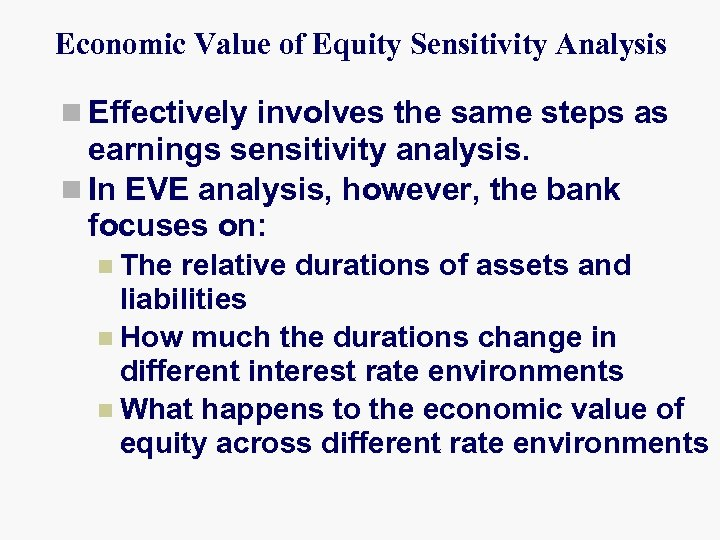Economic Value of Equity Sensitivity Analysis n Effectively involves the same steps as earnings sensitivity analysis. n In EVE analysis, however, the bank focuses on: n The relative durations of assets and liabilities n How much the durations change in different interest rate environments n What happens to the economic value of equity across different rate environments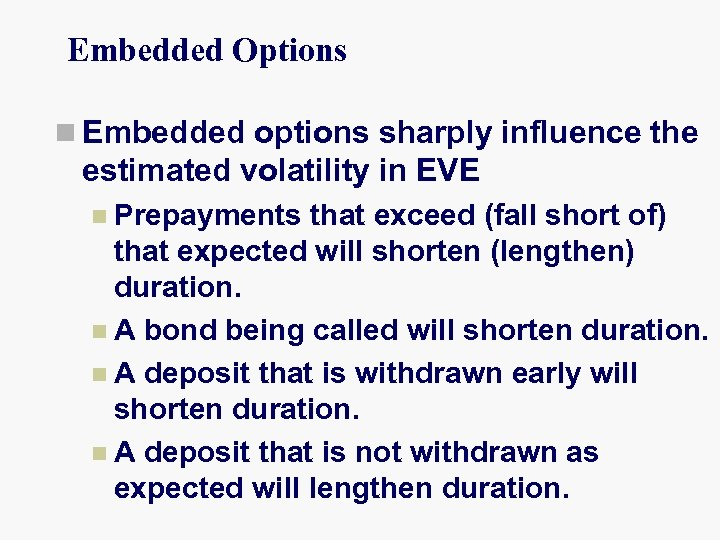Embedded Options n Embedded options sharply influence the estimated volatility in EVE n Prepayments that exceed (fall short of) that expected will shorten (lengthen) duration. n A bond being called will shorten duration. n A deposit that is withdrawn early will shorten duration. n A deposit that is not withdrawn as expected will lengthen duration.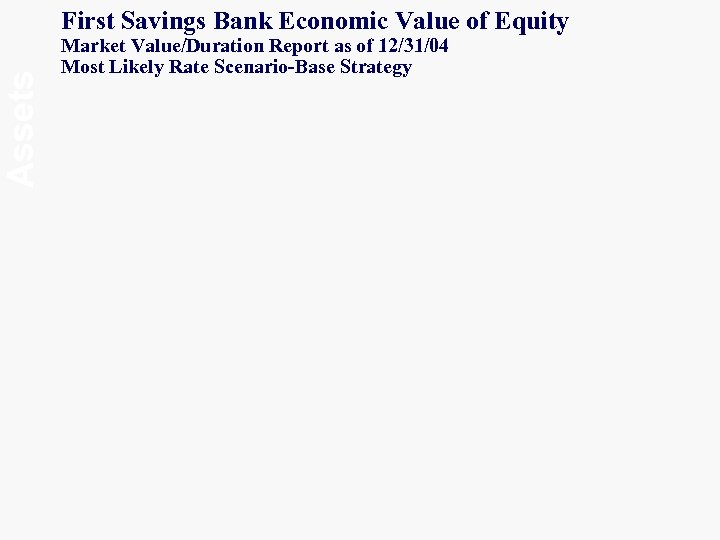Assets First Savings Bank Economic Value of Equity Market Value/Duration Report as of 12/31/04 Most Likely Rate Scenario-Base Strategy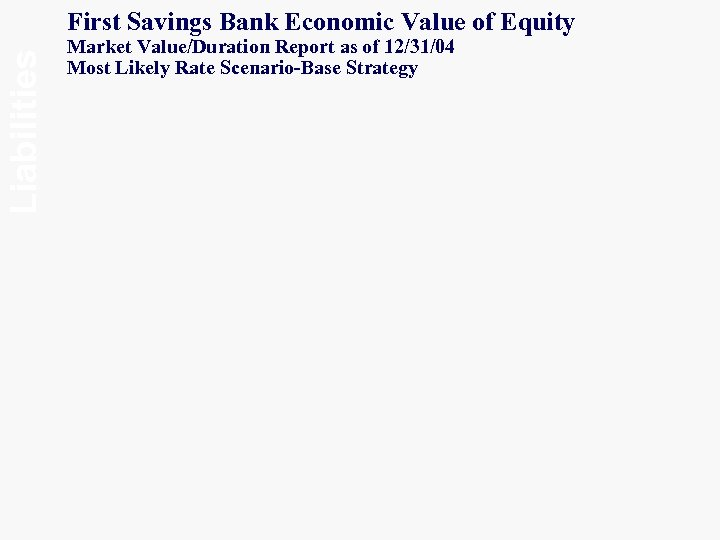Liabilities First Savings Bank Economic Value of Equity Market Value/Duration Report as of 12/31/04 Most Likely Rate Scenario-Base Strategy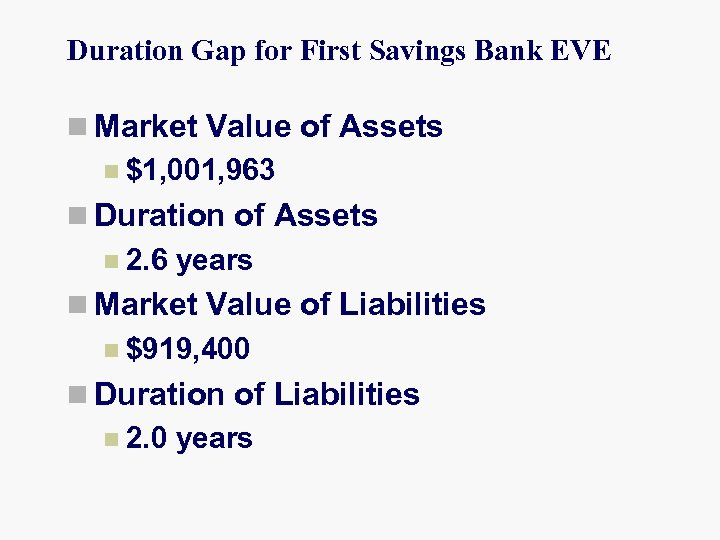Duration Gap for First Savings Bank EVE n Market Value of Assets n \$1, 001, 963 n Duration of Assets n 2. 6 years n Market Value of Liabilities n \$919, 400 n Duration of Liabilities n 2. 0 years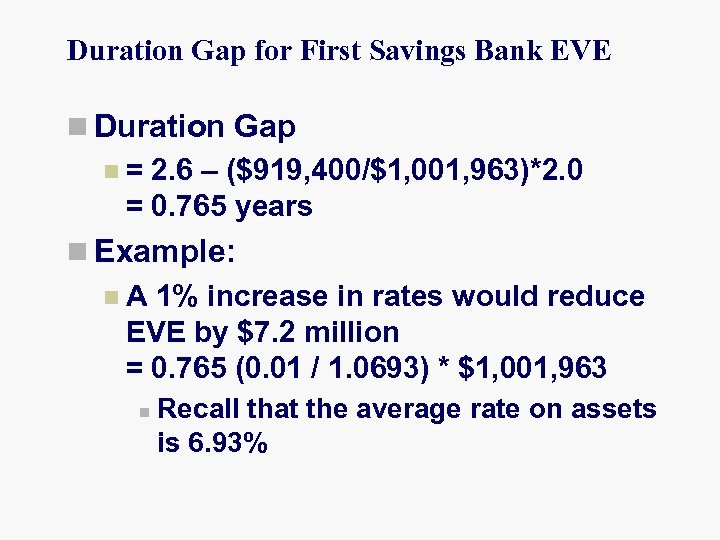Duration Gap for First Savings Bank EVE n Duration Gap n = 2. 6 – (\$919, 400/\$1, 001, 963)*2. 0 = 0. 765 years n Example: n A 1% increase in rates would reduce EVE by \$7. 2 million = 0. 765 (0. 01 / 1. 0693) * \$1, 001, 963 n Recall that the average rate on assets is 6. 93%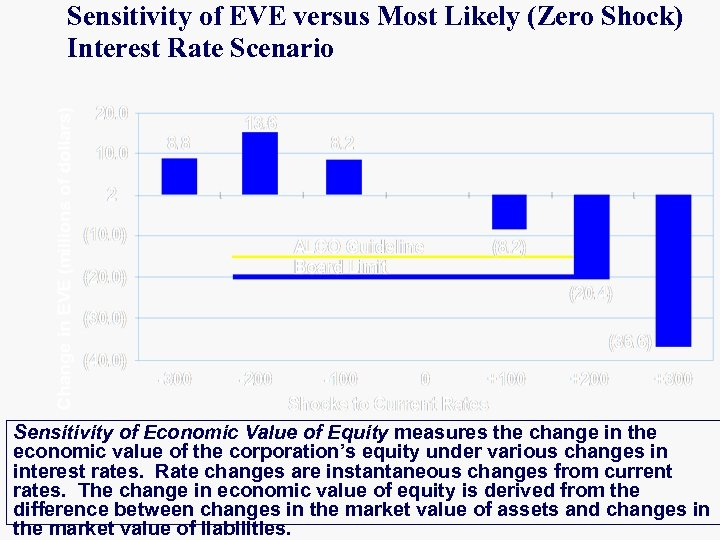Sensitivity of EVE versus Most Likely (Zero Shock) Interest Rate Scenario Sensitivity of Economic Value of Equity measures the change in the economic value of the corporation’s equity under various changes in interest rates. Rate changes are instantaneous changes from current rates. The change in economic value of equity is derived from the difference between changes in the market value of assets and changes in the market value of liabilities.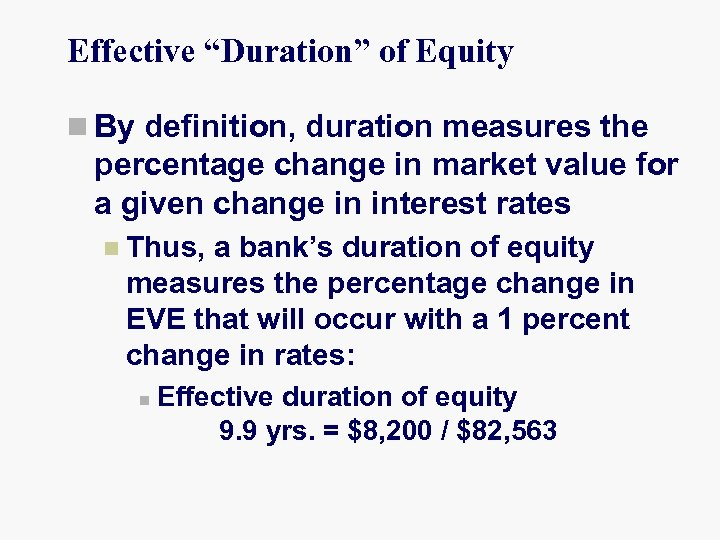Effective “Duration” of Equity n By definition, duration measures the percentage change in market value for a given change in interest rates n Thus, a bank’s duration of equity measures the percentage change in EVE that will occur with a 1 percent change in rates: n Effective duration of equity 9. 9 yrs. = \$8, 200 / \$82, 563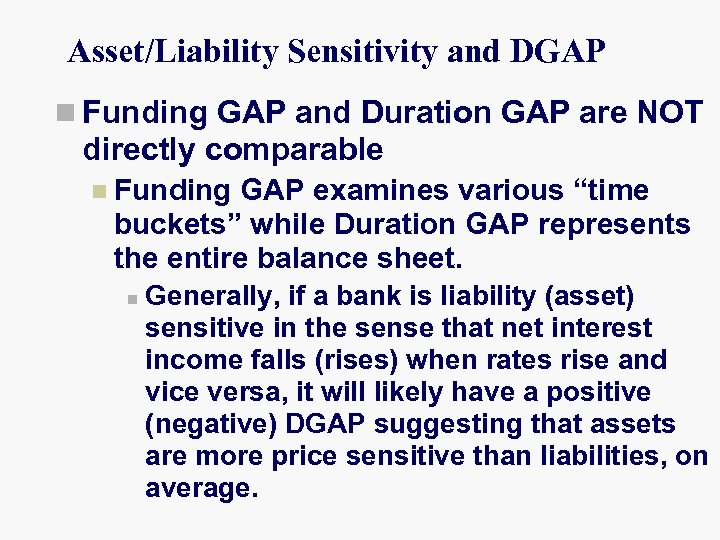Asset/Liability Sensitivity and DGAP n Funding GAP and Duration GAP are NOT directly comparable n Funding GAP examines various “time buckets” while Duration GAP represents the entire balance sheet. n Generally, if a bank is liability (asset) sensitive in the sense that net interest income falls (rises) when rates rise and vice versa, it will likely have a positive (negative) DGAP suggesting that assets are more price sensitive than liabilities, on average.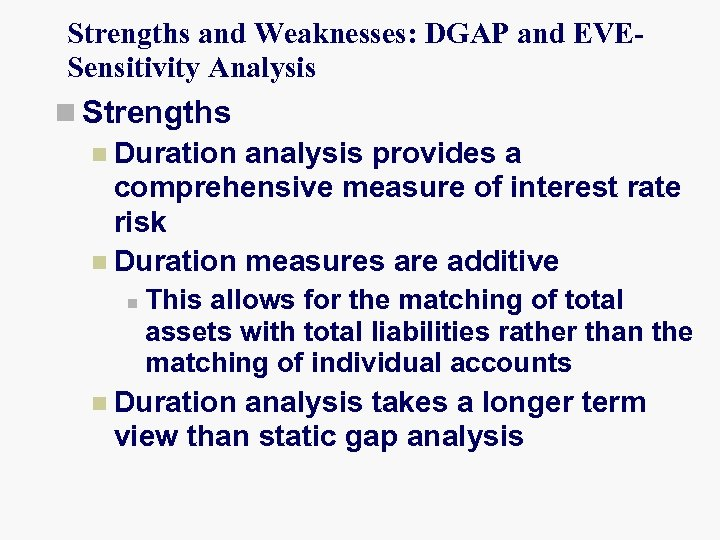Strengths and Weaknesses: DGAP and EVESensitivity Analysis n Strengths n Duration analysis provides a comprehensive measure of interest rate risk n Duration measures are additive n This allows for the matching of total assets with total liabilities rather than the matching of individual accounts n Duration analysis takes a longer term view than static gap analysis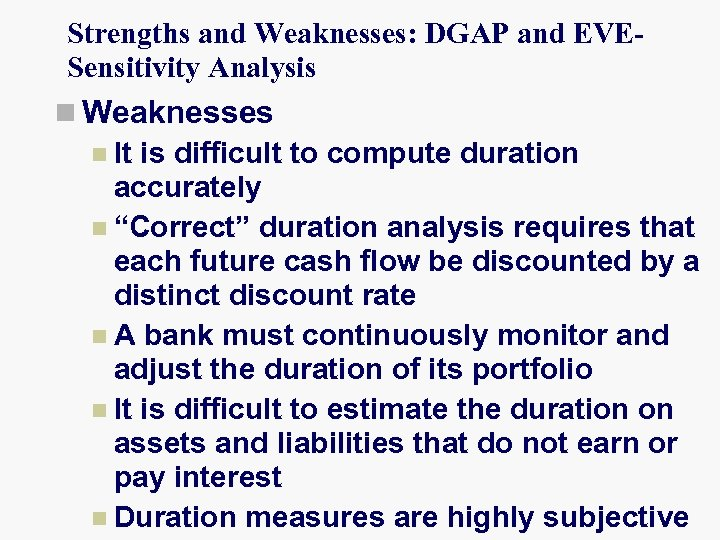Strengths and Weaknesses: DGAP and EVESensitivity Analysis n Weaknesses n It is difficult to compute duration accurately n “Correct” duration analysis requires that each future cash flow be discounted by a distinct discount rate n A bank must continuously monitor and adjust the duration of its portfolio n It is difficult to estimate the duration on assets and liabilities that do not earn or pay interest n Duration measures are highly subjective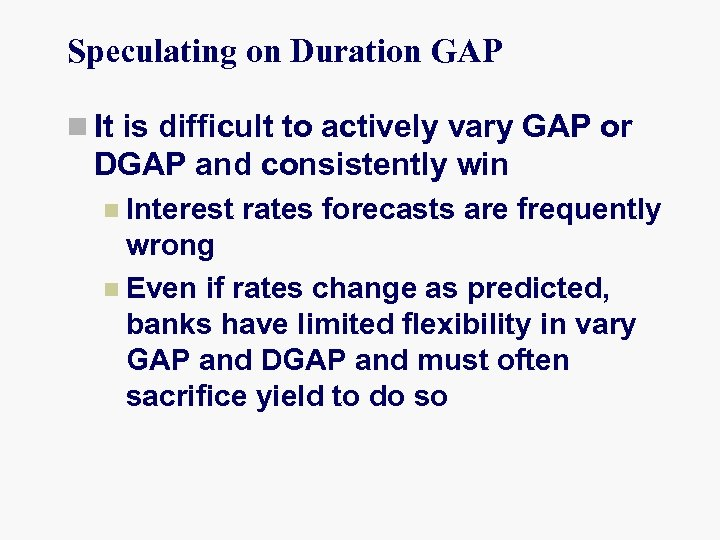Speculating on Duration GAP n It is difficult to actively vary GAP or DGAP and consistently win n Interest rates forecasts are frequently wrong n Even if rates change as predicted, banks have limited flexibility in vary GAP and DGAP and must often sacrifice yield to do so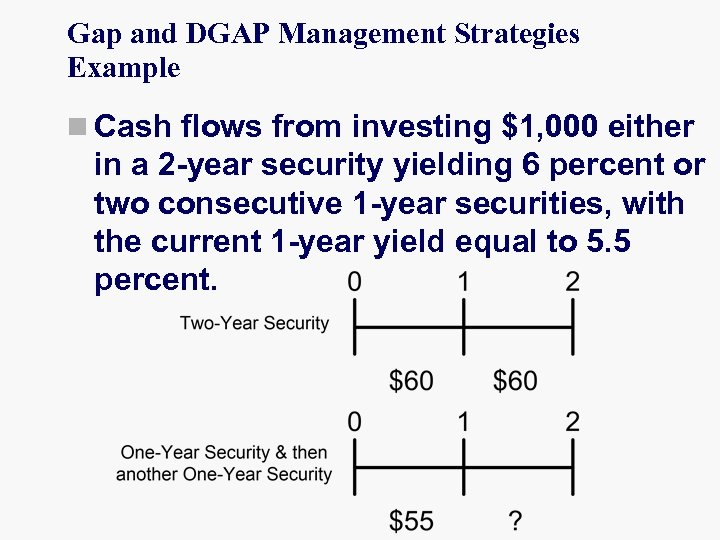Gap and DGAP Management Strategies Example n Cash flows from investing \$1, 000 either in a 2 -year security yielding 6 percent or two consecutive 1 -year securities, with the current 1 -year yield equal to 5. 5 percent.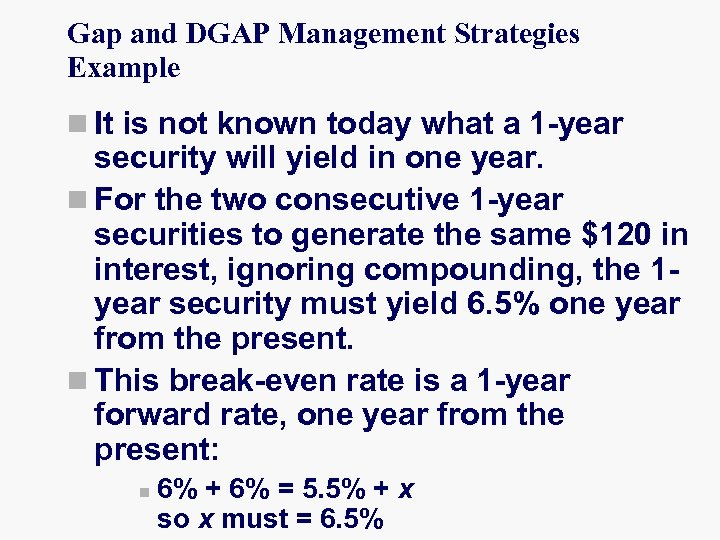Gap and DGAP Management Strategies Example n It is not known today what a 1 -year security will yield in one year. n For the two consecutive 1 -year securities to generate the same \$120 in interest, ignoring compounding, the 1 year security must yield 6. 5% one year from the present. n This break-even rate is a 1 -year forward rate, one year from the present: n 6% + 6% = 5. 5% + x so x must = 6. 5%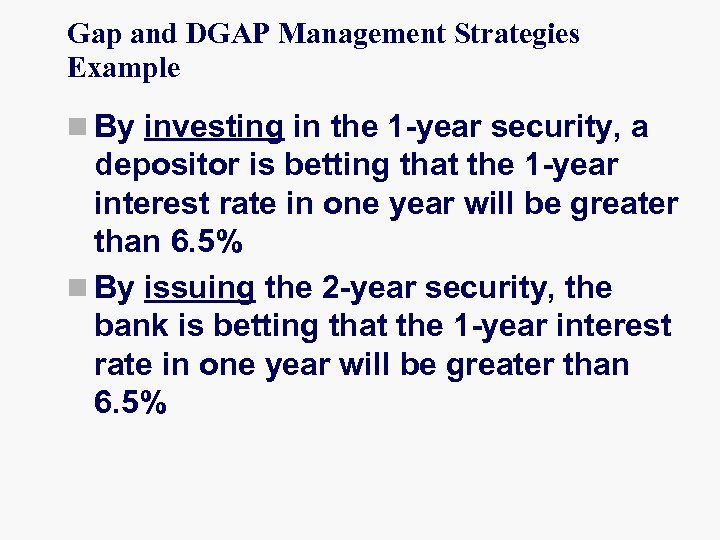Gap and DGAP Management Strategies Example n By investing in the 1 -year security, a depositor is betting that the 1 -year interest rate in one year will be greater than 6. 5% n By issuing the 2 -year security, the bank is betting that the 1 -year interest rate in one year will be greater than 6. 5%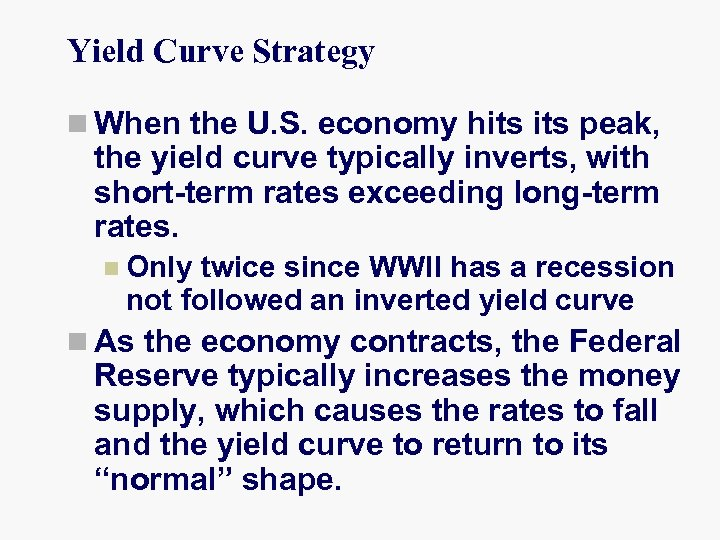Yield Curve Strategy n When the U. S. economy hits peak, the yield curve typically inverts, with short-term rates exceeding long-term rates. n Only twice since WWII has a recession not followed an inverted yield curve n As the economy contracts, the Federal Reserve typically increases the money supply, which causes the rates to fall and the yield curve to return to its “normal” shape.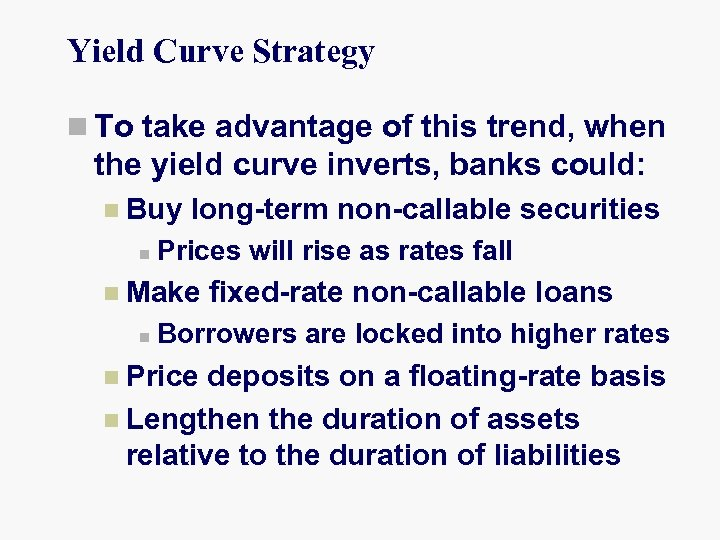Yield Curve Strategy n To take advantage of this trend, when the yield curve inverts, banks could: n Buy n long-term non-callable securities Prices will rise as rates fall n Make n fixed-rate non-callable loans Borrowers are locked into higher rates n Price deposits on a floating-rate basis n Lengthen the duration of assets relative to the duration of liabilities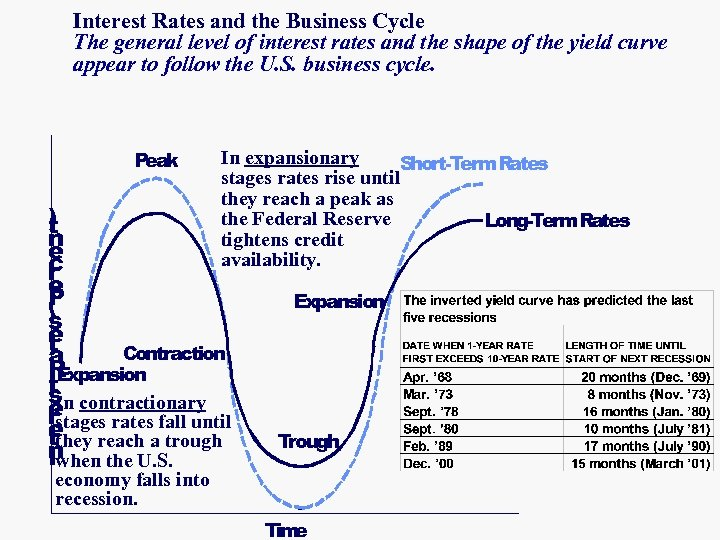Interest Rates and the Business Cycle The general level of interest rates and the shape of the yield curve appear to follow the U. S. business cycle. Peak In expansionary Short-Term. Rates stages rates rise until they reach a peak as the Federal Reserve Long-Term. Rates tightens credit availability. ) t n e c r e P ( s e t Contraction a R t. Expansion s contractionary In e rstages rates fall until e reach a trough tthey n Iwhen the U. S. Expansion Trough economy falls into recession. Tim e